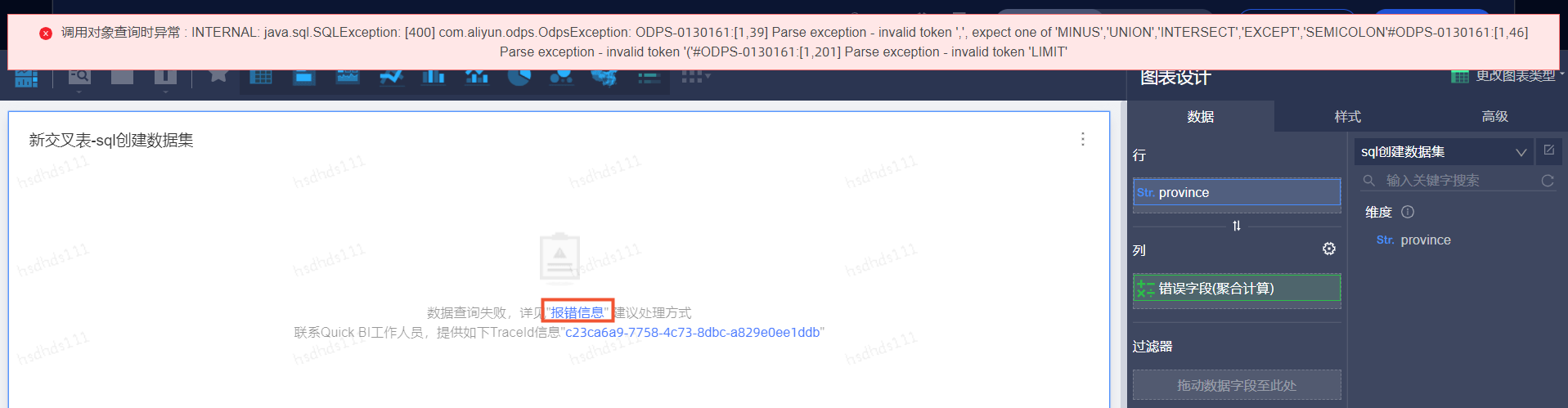# Quick BI报错“调用对象查询时异常：... Parse exception - invalid token...”

## 问题描述## 问题原因

SQL语法有误，需要仔细排查带有聚合函数的计算字段。数据集预览正常，仪表板报错，是因为数据集预览时，不会预览带有聚合函数的字段。

## 解决方案

• 聚合函数后面不写括号
``错误写法：count [字段a]``正确写法：count([字段a])``
• 计算字段引用的字段名不是通过单击右侧字段列表或者键盘点击“[”唤出的，而是手动打上的。
``错误写法：count(字段名)``正确写法：count([字段a])``
• 除法没有考虑分母为0的情况
``错误写法`：sum([字段a])/sum([字段b])正确写法：case when sum([字段b])=0 then 0 else sum([字段a])/sum([字段b]) end`
• 写计算字段时，函数前写“=”
``错误写法`：=count([字段a])正确写法：count([字段a])`

• Quick BI